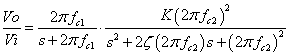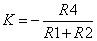# 3rd order Multiple feedback Low-pass Filter Design Tool

This page is a web calculator that design a 3rd order Multiple feedback low-pass filter. Use this utility to simulate the Transfer Function for filters at a given frequency, damping ratio ζ or values of R and C. The response of the filter is displayed on graphs, showing Bode diagram, Nyquist diagram, Impulse response and Step response.

## Calculate the transfer function for 3rd order Multiple feedback low-pass filter with R and C values

 Vi→→Vo
Transfer function:
Transfer function of 3rd order Multiple feedback low-pass filter

2nd order Multiple feedback low-pass filter tool, use also.
 R1=Ω C1=F R2=Ω C2=F R3=Ω C3=F R4=Ω
p:pico, n:nano, u:micro, k:kilo, M:mega

### Frequency analysis

Bode diagram
Phase  Group delay
Nyquist diagram
Pole, zero
Phase margin
Oscillation analysis
Analysis on frequency range:
f1=∼f2=[Hz] (optional)

### Transient analysis

Step response
Impulse response
Overshoot
Final value of the step response
Analysis on time range:
0∼[sec] (optional)

## Calculate the R and C values for the Multiple feedback filter at a given frequency and damping ratio ζ

 Vi→→Vo
※ 3rd order filters are usually made from combinations of 1st and 2nd order filters following the equivalent block diagram.

Equivalent block diagram:

 Vi→ 2πfc1 s+2πfc1 → K(2πfc2)2 s2+2ζ(2πfc2)s+(2πfc2)2 →Vo

Transfer function block diagram:Filter gain at f=0Hz:Filter gain at f=0Hz:
K=[Times] (K<0)

### Select filter type

Set parameters of the equivalent block diagram
 1st order filter: fc1=Hz 2nd order filter: fc2=Hz Damping ratio ζ=

Butterworth filter
Cut-off frequency fc=Hz
Chebyshev filter
Characteristic frequency
fc=Hz
Gain ripple
gr=dB
Bessel filter
Characteristic frequency
fc=Hz

 C1 = F C2 = F
C1, C2 is optional. But when setting these capacitances, C1 and C2 of both are needed.

Select Capacitor Sequence:
Select Resistor Sequence:

### Frequency analysis

Bode diagram
Phase  Group delay
Nyquist diagram
Pole, zero
Phase margin
Oscillation analysis
Analysis on frequency range:
f1=∼f2=[Hz] (optional)

### Transient analysis

Step response
Impulse response
Overshoot
Final value of the step response
Analysis on time range:
0∼[sec] (optional)